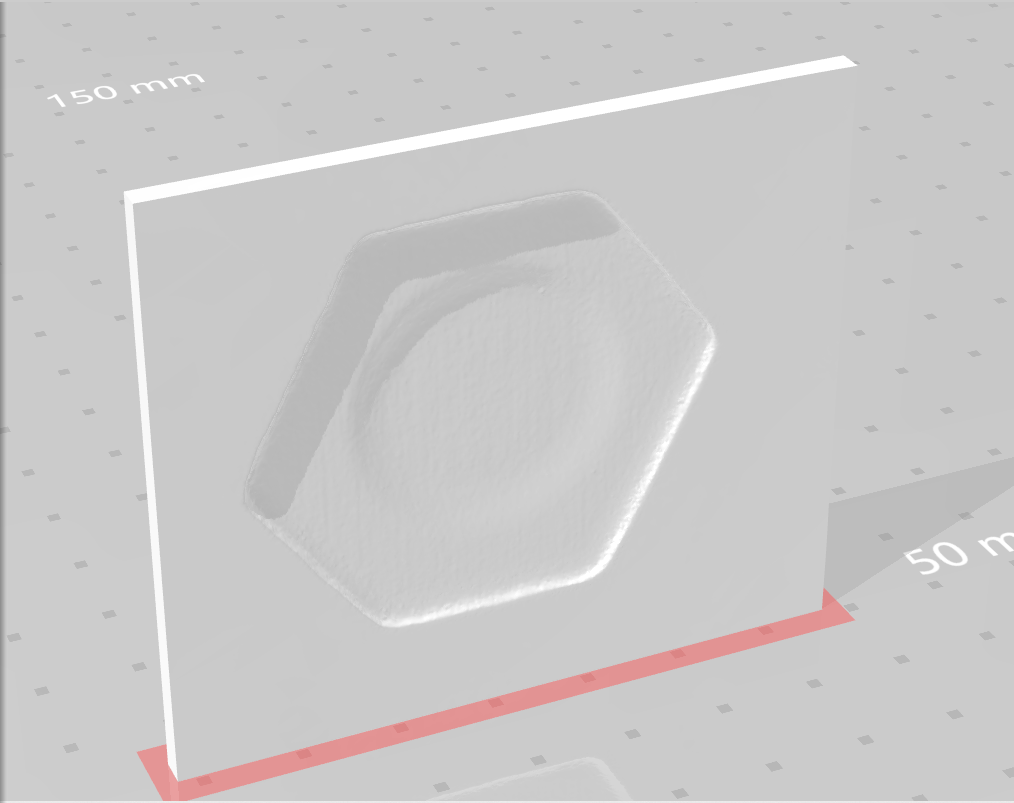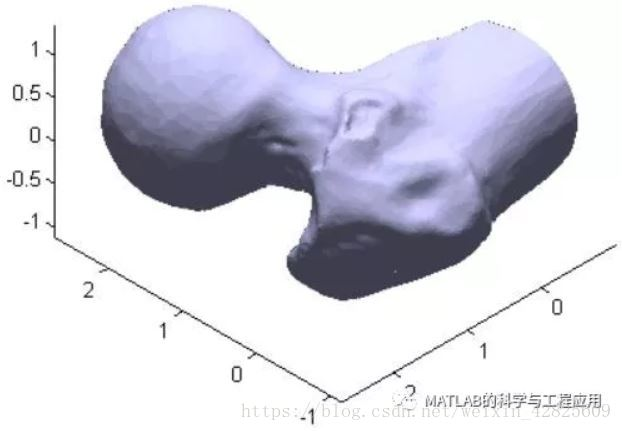• 此程序可以焊好的读取STL文件，可以输出F（面数），V（顶点），N（三角面片的法向量）等，可以稳定输出
• 用于三维光学形貌扫描完成后，生成的stl文件读取，并形成俯视投影云图
• STL文件的基础知识 STL 文件中的数据以三角形曲面网格（也称为三角形镶嵌）的形式存储。 这使得 3D 打印（增材制造）应用的切片变得容易。 要从 STL 文件中读取数据，我们必须了解其中存储数据的格式。STL 文件中...
• MATLAB快速读取STL文件

千次阅读 2020-11-30 21:38:46

HPC_ZY

一、STL文件格式

binary格式ascii格式stl

由于目前没拿到过这种格式的stl，所以暂时不讲

二、开源代码

安装方法

老样子，在附加功能中搜索“Computation of craniofacial symmetry”，看到一个蓝白骷髅头，就是它了。使用方法

% 读取

% 显示
patch('vertices',v,'faces',f,'edgecolor','none',...
'facecolor',[1 0 0],'facelighting','phong')
light
axis equal off

% 保存
stlWrite('newdata.stl',f,v);

三、快速读取

由于MATLAB擅长处理矩阵运算，所以我们采取“先读取，再解析”的方法。

binary格式stl

%% 一、以二进制按字节读取数据
fp = fopen(fileName,'rb');
fclose(fp);

%% 二、提取有效信息
% 提取数据长度信息（四字节无符号整形）
len = typecast(src(81:84),'uint32');

% 提取三角片信息（[48有效字节+2填充字节]*len）
data = reshape(src(85:end),[50,len]);
data(end-1:end,:) = [];

% 类型转换(float*12*len)
dataf = typecast(data(:),'single');
dataf = reshape(dataf,[12,len]);

%% 三、获取v、f、n
% 获取v,f,n（注意MATLAB是列优先的，所以必须按下列方式写）
n = dataf(1:3,:)';
v = reshape(dataf(4:end,:),[3,len*3])';
f = reshape((1:len*3)',[3,len])';

% 去除重复顶点
[v, ~, indexn] =  unique(v, 'rows');
f = indexn(f);

注：比如两个相邻三角片就有重复的定点，而多数原始STL数据并没有去除这些重复定点（这也是导致某些STL数据过大的原因之一）

ascii格式stl

由于目前没拿到过这种格式的stl，所以暂时不讲

四、效果对比

fileName = 'test.stl';
tic
% MATLAB三方
toc
tic
% 个人
toc因为充分利用的MATLAB对矩阵的处理，提速很明显。

其他

欢迎小伙伴提供 ascii格式的stl

展开全文STL 三维模型
• matlabstl文件读取

热门讨论 2013-12-10 20:21:26
• stl文件读取代码，兼容ASCII和二进制类型stl模型文件，网上都是只有二进制类型的，自己写的，测试了几十个3D模型，能用。

笔者前两天帮师兄干了个活，从stl文件里提取金属板亚表面腐蚀缺陷的深度信息，以矩阵的形式给他。

模型如图所示亚表面（即背部）由于腐蚀缺陷出现凹坑，要提取其深度信息。千辛万苦终于弄明白，特此在这里记录一下。

软件工具：matlab

官方文档基本说的很详细了TR = stlread(filename) 返回 triangulation 对象TR，其中包含 STL 文件中的三角剖分数据

triangulation的官方文档https://ww2.mathworks.cn/help/matlab/ref/triangulation.html这里用到 triangulation类的内置函数Points，获取对象TR中三角剖分的顶点坐标数据，matlab语句为

XYZ=TR.Points;

由于顶点数据是无序的，需要筛选出位于亚表面的顶点以计算缺陷深度。完整程序如下：

%%%%%%%%%%%%%%%%%%%%%%%%%%%%%%%%%%%%%%%%%%%%%%%%%%%%%%%%%%%%%%%

%优化了扫查速度
clear;
tic;
XYZ=TR.Points;%板的法向沿y方向
%步长,受stl精细度限制
step=0.4;
%初始点
xstart=min(XYZ(:,1));
zstart=min(XYZ(:,3));
%矩阵大小,向下取整
xnums=floor((max(XYZ(:,1))-min(XYZ(:,1)))/step);
znums=floor((max(XYZ(:,3))-min(XYZ(:,3)))/step);
%坐标序列
xseries=xstart:step:max(XYZ(:,1));
zseries=zstart:step:max(XYZ(:,3));
%初始化矩阵
Depth=zeros(xnums,znums);
%以扫查步长内的最小y坐标作为作为该点的亚表面y坐标
hh = waitbar(0,'Searching...');
counter=0;
xflag=1;
xsort=sortrows(XYZ,1);
for cntx=1:xnums
xend=find(xsort(xflag:end,1)>xseries(cntx+1),1)+xflag-2;
zflag=1;
zsort=sortrows(xsort(xflag:xend,:),3);
for cntz=1:znums
zend=find(zsort(zflag:end,3)>zseries(cntz+1),1)+zflag-2;
Depth(cntx,cntz)=min(zsort(zflag:zend,2));
zflag=zend+1;
counter=1+counter;
waitbar(counter/(xnums)/(znums))
end
xflag=xend+1;
end
close(hh);
toc;
%深度
Depth(:,:)=Depth(:,:)-min(min(Depth));
%作图
zseries=zseries(1:end-1);
xseries=xseries(1:end-1);
surfc(zseries,xseries,Depth)
alpha 0.8
xlabel('X (mm) ')
ylabel('Y (mm) ')
zlabel('Depth (mm)')
title('亚表面缺陷深度分布')

%%%%%%%%%%%%%%%%%%%%%%%%%%%%%%%%%%%%%%%%%%%%%%%%%%%%%%%%%%%%%%%

其中“18.stl”是位于程序同目录下的stl文件。

大概就这么多吧，欢迎大家参考~

展开全文matlab stl
• Matlab读取并输出stl文件

千次阅读 2020-03-03 11:45:58
*#利用matlab读取stl文件后，将其中三角形片数据×2后，保存到另一个stl文件，利用3D软件打开观察图形是否变为两倍。那么应该怎么做呢？ 首先了解一下stl文件 STL(Stereo lithographic)文件格式是美国3D SYSTEMS...

*#利用matlab读取stl文件后，将其中三角形片数据×2后，保存到另一个stl文件，利用3D软件打开观察图形是否变为两倍。那么应该怎么做呢？

首先了解一下stl文件
STL(Stereo lithographic)文件格式是美国3D SYSTEMS公司提出的三维实体造型系统的一个接口标准，其接口格式规范。采用三角形面片离散地近似表示三维模型，目前已被工业界认为是快速成形(rapid prototypi ng)领域的标准描述文件格式。在逆向工程、有限元分析、医学成像系统、文物保护等方面有广泛的应用。

STL文件的最大特点也是其主要问题是，它是由一系列的三角形面片无序排列组合在一起的，没有反映三角形面片之间的拓扑关系。

stl文件分为二进制文件和ASCII文件两种：

二进制STL文件用固定的字节数来给出三角面片的几何信息。文件起始的80个字节是文件头，用于存贮零件名；紧接着用4个字节的整数来描述模型的三角面片个数，后面逐个给出每个三角面片的几何信息。每个三角面片占用固定的50个字节，依次是3个4字节浮点数(角面片的法矢量)，3个4字节浮点数(1个顶点的坐标)，3个4字节浮点数(2个顶点的坐标)，3个4字节浮点数(3个顶点的坐标)，最后2个字节用来描述三角面片的属性信息。一个完整二进制STL文件的大小为三角形面片数乘以50再加上84个字节，总共1 34个字节。

ASCII码格式的STL文件逐行给出三角面片的几何信息，每一行以1个或2个关键字开头。在STL文件中的三角面片的信息单元facet是一个带矢量方向的三角面片，STL三维模型就是由一系列这样的三角面片构成。整个STL文件的首行给出了文件路径及文件名。在一个STL文件中，每一个facet由7行数据组成，facetnormal是三角面片指向实体外部的法矢量坐标，outer loop说明随后的3行数据分别是三角面片的3个顶点坐标，3顶点沿指向实体外部的法矢量方向逆时针排列。

话不多说，直接上代码

读取ASCII文件：

clc;
t=cputime;
filefrom='城堡-ASCLL.stl';
fid1=fopen(filefrom);
fgetl(fid1);
data=fscanf(fid1,'  facet normal %e %e %e\n    outer loop\n    vertex %e %e %e  vertex %e %e %e  vertex %e %e %e\n  endloop\n  endfacet\n');
fileto='城堡-ASCLL2.stl';
[length, w] = size(data);
fid2 = fopen(fileto, 'w');
data = data.*2;
fprintf(fid2, "solid 城堡-ASCLL2\n");
for i=1:length / 12
fprintf(fid2, '  facet normal %e %e %e\n        outer loop\n            vertex %e %e %e\n           vertex %e %e %e\n           vertex %e %e %e\n       endloop\n  endfacet\n', ...
data((i - 1) * 12 + 1:i* 12, 1) );
end
fprintf(fid2, "endsolid\n");
fclose(fid1);
fclose(fid2);
e=cputime-t;
disp('耗时为：');
disp(e);

读取二进制stl文件：

clear;
clc;
starttime = tic;
% 读取和写入的文件名
filenamefrom='城堡-二进制.stl';
filenameto = '城堡-二进制2.stl';

% 以二进制打开文件读取原文件
fid1 = fopen(filenamefrom, 'rb');
fid2 = fopen(filenameto, 'wb');

% 读出表头写入文件
fwrite(fid2, data, 'uint8');

% 读出三角矩阵的个数
fwrite(fid2, num, 'uint32');

% 将个数值翻倍之后，写入num个三角矩阵
for i=1:num
vector = vector.*2;
fwrite(fid2, vector, 'float');
fwrite(fid2, attribute, 'uint16');
end

% 关文件
fclose(fid1);
fclose(fid2);

% 结束计时
timeelapsed = toc(starttime);
disp("运行时间:");
disp([num2str(timeelapsed),'s']);

展开全文matlab stl
• 立体光刻(STL)文件是一种用于存储网格数据的通用格式，STL网格只是三角形面的集合。这种类型的模型非常适合与MATLAB的PATCH图形对象一起使用。% Import an STL mesh, returning a PATCH-compatible face-vertex ...

本示例对人体股骨的3D模型进行加载并渲染，展示了MATLAB的一些高级图形处理特点，包括照明和镜面反射。

立体光刻(STL)文件是一种用于存储网格数据的通用格式，STL网格只是三角形面的集合。这种类型的模型非常适合与MATLAB的PATCH图形对象一起使用。

% Import an STL mesh, returning a PATCH-compatible face-vertex structure

用PATCH图形对象渲染模型。我们还添加了一些动态照明，并调整材料属性以改变镜面强调照射的效果。

patch(fv,‘FaceColor’, [0.8 0.8 1.0], …

'EdgeColor', 'none', ...

'FaceLighting', 'gouraud', ...

'AmbientStrength', 0.15);

% Add a camera light, and tone down the specular highlighting

material(‘dull’);

% Fix the axes scaling, and set a nice view angle

axis(‘image’);

view([-135 35]);部分MATLAB代码：

% STLREAD imports geometry from an STL file into MATLAB.

% FV = STLREAD(FILENAME) imports triangular faces from the ASCII or binary

% STL file idicated by FILENAME, and returns the patch struct FV, with fields

% ‘faces’ and ‘vertices’.

%

% [F,V] = STLREAD(FILENAME) returns the faces F and vertices V separately.

%

% [F,V,N] = STLREAD(FILENAME) also returns the face normal vectors.

%

% The faces and vertices are arranged in the format used by the PATCH plot

% object.

% Copyright 2011 The MathWorks, Inc.

if ~exist(file,'file')

error(['File ''%s'' not found. If the file is not on MATLAB''s path' ...

', be sure to specify the full path to the file.'], file);

end

fid = fopen(file,'r');

if ~isempty(ferror(fid))

error(lasterror); %#ok

end

fclose(fid);

[f,v,n] = stlbinary(M);

完整MATLAB下载地址：

http://page2.dfpan.com/fs/8lcj9221e291b6ce395/

更多精彩文章请关注微信号：

展开全文• HYDRAULICS Mar．2018 Vo1．46 No．5 DOI：10．3969／j．issn．1001—3881．2018．05．025 一 种基于 MATLABSTL文件分层切 片算法 丁华锋 ，王卓 ，刘婧芳 ，孙龙 ，张良安 (1．北京工业大学机械 工程及应用电子...
• 2018 3 Mar.2018 46 5 MACHINE TOOL ＆ HYDRAULICS Vol.46 No.5 ： 10.3969／j.issn.1001－3881.2018.05.025 MATLAB STL 1 1 1 2 2 ， ， ， ， （ 1.， 100124； 2.， 243032 ） ： STL 3D 。 ， MAT－ LAB STL ， ...
• 读取包含顶点和面的 ASCII STL 文件，并将其结构化为矩阵“顶点”、“颜色”和... 我使用 C++ mex 函数读取 STL 文件，因此它的运行速度比在 matlab 函数上读取要快得多。 它建立在 MacOS 版本 10.14.5 上MATLAB 2018amatlab
• Matlab读取显示STL\OBJ文件

万次阅读 热门讨论 2018-05-06 13:26:46
最近在做图形学的一些任务，其中有一部分是使用MATLAB读取OBJ、STL、OFF文件。 其实OFF和OBJ很相似，所以这里只给出OBJ的文件和STL文件的读取。 最近抽空整理一下。 说明 我的环境时Macos+matlab r2014b 对于...matlab obj stl off
• matlab如何读取并显示stl模型

千次阅读 2020-12-27 19:45:06
• function [VertexData,FVCD,isBinary]=stl2matlab(stlfile) % STL2MATLAB reads STL-file, ASCII or binary format, into Matlab % % Usage: % % [VertexData, FVCD]=stl2matlab(stlfile) % % Input: % %.....
• FV = STLREAD(FILENAME) 从二进制 STL 文件导入三角面由 FILENAME 指示，并返回补丁结构 FV，带有字段“faces” 和“顶点”。 [F,V] = STLREAD(FILENAME) 分别返回面 F 和顶点 V。 [F,V,N] = STLREAD(FILENAME) ...matlab
• MATLAB 中获取有关 STL 文件的信息。 将其用作， [F, V, N] = read_stl_file('myfile.stl'); 其中 F 是“面”，V 是“顶点”，N 是“法线”。 可以绘制对象， p = patch('Faces', F, 'Vertices', V, '...matlab
• 吴建 吴婷 陈廷豪 包涵摘 要：为提高STL模型的切片效率，提出一种基于MATLABSTL模型切片分层新算法。首先对STL模型进行数据预处理，筛选出只与切平面相交的三角片集合，然后利用相邻三角面片边的拓扑关系依次求交...
• 1、读取stl文件 2、将高度分段，例如分成20段 3、根据段确定彩色条范围，并且画出点。 （*这个案例里面没有插值，也没有根据stl文件均匀地求解高度点，仅仅只是拿原文件的数据点以不同颜色显示*） ...matlab 图像处理 开发语言
• 资源包含了用c++和matlab分别读取、写入和修改.stl文件，欢迎大家下载！ 另附大量sw模型和.stl文件matlab
•可视化
• 一、文件读取 文件保存成csv,或者txt，逗号分割； res = readtable('/User/xxx/path/file.csv') size = height(res) 二、绘制曲线 figure(1) plot(res.x1(start_index:end_time),res.y1(start_index:end_time)...matlab
• 使用matlab 读取展示3D模型必备软件！！！！matlab stl
• 如何同patch来可视化我读取stl文件呢， [f,v,n] = stlread('TEST_STL.stl'); patch(f,v,n) 代码如上，但是总是报错： Error using patch Not enough input arguments. 我查了很多方法用patch都是这样报错matlab 计算机视觉 人工智能
• 原模型如下： 从mathworks下载stltools工具包：...%读取文件格式是binary还是ascii stlGetFormat('dentition.stl') %选择相应的读取器 [v, f, n, name] = stlReadBi...MATLAB...

matlab读取stl文件matlab 订阅